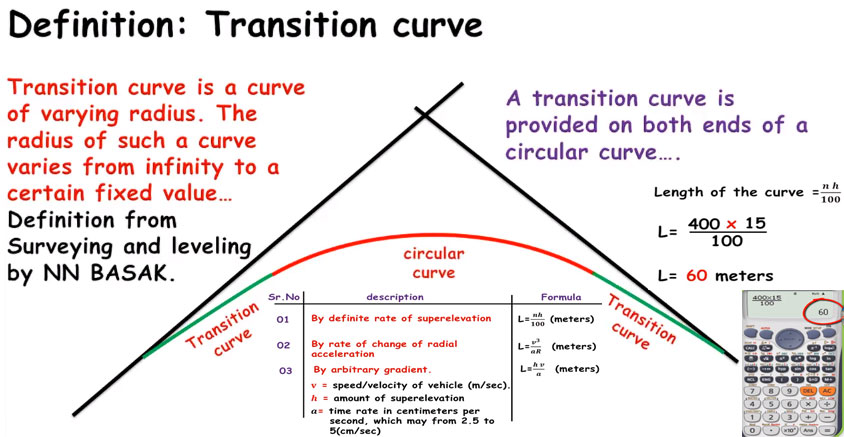# How to determine the length of a transition curveIn this construction video tutorial, you will learn how to determine the length of a transition curve by applying various methods like definite rate of superelevation, rate of change of radial acceleration and arbitrary gradient.

Definition of transition curve may be given as following :-

The transition curve stands for a horizontal curve with inconstant radius that is applied to attach straight line to circular curve. The radius of curve changes from infinite to certain fix value.

Normally, transition curve is arranged at both ends of the circular curve.

Occasionally, it is also arranged at end of compound curve. It is very effective for transportation engineer, highway engineering and surveyors.

Benefits of transition curve: If there is no transition curve, the risk for overturning of train or truck may occur. This vehicle can skid and leads to accidents on road.

Transition curve contributes to sluggish increase of curvature that facilitates a driver to increase the speed of vehicle. It also allows the vehicle to drive at greater speed in curve.

The following properties should be included in a curve to be qualified as a transition curve :-

• It's radius should have been infinite at origin on the tangent.
• Where the circular curve coincides at that curve, on that point radius must be equivalent with the radius of circular curve.
• There should not be any divergence among rate of increase of super elevation and the rate of increase of curvature.

The formula of definite rate of superelevation is L = nh/100 (meters)

Where, h denotes superelevation in centimetres and L denotes length of transition curve in meters.

The definite rate of superelevation should be assumed as 1 in n.

The formula of rate of change of radial acceleration is L = v3/ar (meters)

Where, v = speed/velocity of vehicle (m/sec)
a = rate of radial acceleration
L = length of transition curve in meters

The formula of arbitrary gradient is L = hv/a (meters)

Where, v = speed/velocity of vehicle (m/sec)

h = amount of superelevation
a = time rate in centimetres per second

To gather more details, go through the following video tutorial.

Video Source: SL Khan